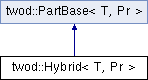# twod::Hybrid< T, Pr > Class Template Reference

hybrid algorithms for 2d partitioning. A first partitioning is done with a 2d algorithm. Then, each part is partitioned during a second level using an other algorithm. More...

`#include <twod/hybrid.hpp>`

Inheritance diagram for twod::Hybrid< T, Pr >:## Detailed Description

### template<typename T, typename Pr> class twod::Hybrid< T, Pr >

hybrid algorithms for 2d partitioning. A first partitioning is done with a 2d algorithm. Then, each part is partitioned during a second level using an other algorithm.

At first level, the number of processors used at the first level is variable (but can be fixed manually using setProcLevel1()). At second level, the processors are distributed among the parts proportionnally to their load (using util::distribute_processors).

The algorithm at level 1 is selected using setLevel1().

To obtain better quality tradeoff, one can use two algorithms at the second phase. Select the fast algorithm using setLevel2() and the slow algorithm using setLevel2Slow(). The slow algorithm will only be used if the fast algorithm does not gets a good load balance.

Parameters:
 T type of the load Pr type of the prefix sum array

List of all members.

## Public Member Functions

void setLevel1 (twod::PartBase< T, Pr > *l1)
set the algorithm at the first level.
void setProcLevel1 (int s1)
set the number of parts to generate at the first level.
void setLevel2 (twod::PartBase< T, sPr > *l2)
set the algorithm at the second level.
void setLevel2Slow (twod::PartBase< T, sPr > *l2)
set the algorithm at the second level for the difficult case.
virtual T part (int procCount, const Pr &prefixSumArray, util::RectList< T, Pr > &parts)
virtual ~Hybrid ()

## Private Types

typedef util::SubPSA< T, PrsPr

## Private Member Functions

void probephase1 (int procCount, const Pr &prefixSumArray, util::RectList< T, Pr > &l1)
int better_guess (int p, int m)
this function returns the maximum p' such that ceil(m-p')/p' = ceil(m-p)/p
void phase1 (int procCount, const Pr &prefixSumArray, util::RectList< T, Pr > &l1)
void phase2 (int procCount, const Pr &prefixSumArray, util::RectList< T, Pr > &parts, const util::RectList< T, Pr > &l1)

## Private Attributes

std::auto_ptr< twod::PartBase
< T, Pr > >
algoLevel1
int s1
std::auto_ptr< twod::PartBase
< T, sPr > >
algoLevel2
std::auto_ptr< twod::PartBase
< T, sPr > >
algoLevel2Slow

## Member Typedef Documentation

template<typename T , typename Pr >
 typedef util::SubPSA twod::Hybrid< T, Pr >::sPr` [private]`

## Constructor & Destructor Documentation

template<typename T , typename Pr >
 twod::Hybrid< T, Pr >::~Hybrid ( ) ` [virtual]`

## Member Function Documentation

template<typename T , typename Pr >
 int twod::Hybrid< T, Pr >::better_guess ( int p, int m ) ` [private]`

this function returns the maximum p' such that ceil(m-p')/p' = ceil(m-p)/p

template<typename T , typename Pr >
 T twod::Hybrid< T, Pr >::part ( int procCount, const Pr & prefixSumArray, util::RectList< T, Pr > & parts ) ` [virtual]`

Applies 2 level hybrid partitioning

Parameters:
 [in] procCount is the number of processors [in] prefixSumArray first column and first row consists of zero only. But the borders of rectangles in rect_list never touch this area (index 0 in row or column) [out] parts must be allocated before calling this function. List of rectangles that form the partition.
Returns:

Implements twod::PartBase< T, Pr >.

template<typename T , typename Pr >
 void twod::Hybrid< T, Pr >::phase1 ( int procCount, const Pr & prefixSumArray, util::RectList< T, Pr > & l1 ) ` [private]`
template<typename T , typename Pr >
 void twod::Hybrid< T, Pr >::phase2 ( int procCount, const Pr & prefixSumArray, util::RectList< T, Pr > & parts, const util::RectList< T, Pr > & l1 ) ` [private]`
template<typename T , typename Pr >
 void twod::Hybrid< T, Pr >::probephase1 ( int procCount, const Pr & prefixSumArray, util::RectList< T, Pr > & l1 ) ` [private]`
template<typename T , typename Pr >
 void twod::Hybrid< T, Pr >::setLevel1 ( twod::PartBase< T, Pr > * l1 )

set the algorithm at the first level.

Take care, the algorithm is passed through a pointer to an object. Hybrid WILL deallocate it (through a std::auto_ptr).

template<typename T , typename Pr >
 void twod::Hybrid< T, Pr >::setLevel2 ( twod::PartBase< T, sPr > * l2 )

set the algorithm at the second level.

Take care, the algorithm is passed through a pointer to an object. Hybrid WILL deallocate it (through a std::auto_ptr).

template<typename T , typename Pr >
 void twod::Hybrid< T, Pr >::setLevel2Slow ( twod::PartBase< T, sPr > * l2 )

set the algorithm at the second level for the difficult case.

Take care, the algorithm is passed through a pointer to an object. Hybrid WILL deallocate it (through a std::auto_ptr).

template<typename T , typename Pr >
 void twod::Hybrid< T, Pr >::setProcLevel1 ( int s1 )

set the number of parts to generate at the first level.

If -1 is selected, the number of parts at the first level is automatically selected by running the first level multiple times and computing an expected gain at level 2.

Note: -1 is the default value.

## Member Data Documentation

template<typename T , typename Pr >
 std::auto_ptr > twod::Hybrid< T, Pr >::algoLevel1` [private]`
template<typename T , typename Pr >
 std::auto_ptr > twod::Hybrid< T, Pr >::algoLevel2` [private]`
template<typename T , typename Pr >
 std::auto_ptr > twod::Hybrid< T, Pr >::algoLevel2Slow` [private]`
template<typename T , typename Pr >
 int twod::Hybrid< T, Pr >::s1` [private]`

The documentation for this class was generated from the following files: